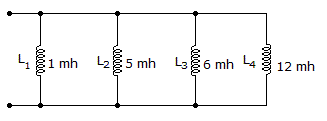# Electronics - Inductors - Discussion

### Discussion :: Inductors - General Questions (Q.No.85)

85.

What is the total inductance assuming no mutual inductance?[A]. 0 mH [B]. 0.69 mH [C]. 12 mH [D]. 24 mH

Explanation:

No answer description available for this question.

 Savitha U.R said: (Apr 16, 2012) Total inductance in parallel(LT) 1/LT=1/L1+1/L2+1/L3+1/L4 =(1/1+1/5+1/6+1/12)1/10^-3 =(1+0.2+0.1667+0.0175)10^3 1/LT=1.3842*10^3 LT=1/(1.3842*10^3) =0.7224*10^-3 =0.72mH~~0.69mH SO Ans is B.

 Shyam Verma said: (Jun 28, 2014) Total inductance in parallel(LT). 1/LT = 1/L1+1/L2+1/L3+1/L4. 1/87/60. 60/86 = 0.69mh.